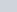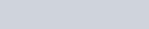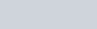# Capacitance formula derivation of parallel plate capacitor with dielectric slab inserted of thickness t.

• 2
•
• 2
•
•
•
•
•
4
Shares

Well, In this article, we are going to derive the expression for capacitance of parallel plate capacitor when a dielectric slab of thickness t is inserted between the plates. Wants to know all about the derivation, stay tuned with us till end…

# DERIVATION FOR THE CAPACITANCE

Let’s take a parallel plate capacitor having plates surface area is A. A dielectric slab of thickness t is inserted between the plates. And the dielectric slab have the dielectric constant K and the permittivity of it’s medium is ε. See figure below :

After putting dielectric slab of thickness t the remaining distance will be (d – t). Let the electric field inside the dielectric slab is E and field inside the plates and outside the slab is.

## DERIVATION PROCESS

The electric field inside the slab is given as-And the electric field outside the slab is given as –The total potential difference between the plates is given as the sum of the potential difference due to the slab and due to without slab. Which is clearly written below :Capacitance of any capacitor is given as charge per unit potential difference. Let’s put the value of potential difference in the formula of capacitance.This is the required value of capacitance of parallel plate capacitor when a dielectric slab of thickness t is inserted between the plates.

• 2
•
• 2
•
•
•
•
•
4
Shares

### One comment

1. #### disgraces

Normallү I don’t read artіcle on blogs, howеver I would like to say that this wｒite-up very forced
me to try and do so! Your writing style has been surprised me.
Thank you, quitе nice article.